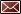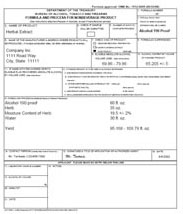Get Email Updates Back to Nonbeverage Products Laboratory page

# Drawback Tutorial

Example formulasDietary Supplements 2 This dietary supplement is made with a wet herb with a known moisture content. Step 1.  Item 9 Calculation – all of the eligible alcohol comes from 190 proof ethanol.  Calculate the volume of absolute alcohol: 80 fl oz. × 0.95 = 76 fl oz. of absolute alcohol Next, divide by the yield.  Low end of yield range – 76 fl oz. ÷ 95.168 fl oz. = 0.7986 or 79.86% High end of yield range – 76 fl oz. ÷ 108.79 fl oz. = 0.6986 or 69.86% Click on the image to enlarge

Step 2.  Item 10 Calculation – This is should be done in a similar way as extracts are calculated.

The volume of absolute alcohol is calculated the same way as in step 1.  The volume of absolute alcohol is then divided by the starting menstruum (volume of ethanol, water and other dissolved components).

In this example, the starting menstruum is ethanol, the water added and the water from the wet herb.

Using the moisture content, the volume of water contributed by the herb can be determined.

35 wt oz. (weight of herb) × 0.195 (moisture content) = 6.825 wt oz. of water from herb
6.825 wt oz. = 6.555 fl oz. water from herb

Starting menstruum:
80 fl oz. (ethanol) + 30 fl oz. (water) + 6.555 (water from herb) = 116.555 fl oz.

76 fl oz. ÷ 116.555 fl oz. = 0.6521 or 65.21%

The tolerance used for dietary supplements for item 10 is always +/- 5.

Note:  The alcohol content of the finished product cannot be less than zero or higher than the theoretical alcohol content (e.g. if 190 proof alcohol is used, the alcohol content of the product cannot be greater than 95%).